# Examples for 5th grade (the fifth graders) - page 22

1. Density 2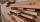What is the density of a piece of driftwood that has the mass of 25 grams and a volume is 30 cm to the 3rd power. And how do I figure it out?
2. The average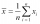The average of one set of 4 numbers is 35. The average of another set of number is 20. The average of the numbers in the two sets is 30. How many numbers are there in the other set?
3. Voting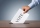400 employees cast their votes in a board member election that has only 2 candidates. 120 people vote for candidate A, while half of the remaining voters abstain. How many votes does candidate B receive?
4. A koala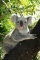A koala lives to be 14 years old in the wild. How much longer will a 2 year old koala probably live?
5. The smallest numberWhat is the smallest number that can be divided by both 5 and 7
6. Cake 71/3 of a cake shared with 4 people. What share of the whole cake has each people?
7. Find the 6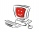Find the total cost of 10 computers at \$ 2100 each and 7 boxes of diskettes at \$12 each
8. DozenWhat is the product of 26 and 5? Write the answer in Arabic numeral. Add up the digits. How many of this is in a dozen? Divide #114 by this
9. Conference 29 people attended a conference on behalf of their company. The conference fee was £520 per person and the company paid a total of £856 in travel costs for the 9 people. How much did the conference cost the company altogether?

We apologize, but in this category are not a lot of examples.
Do you have an interesting mathematical example that you can't solve it? Enter it, and we can try to solve it.

To this e-mail address, we will reply solution; solved examples are also published here. Please enter e-mail correctly and check whether you don't have a full mailbox.